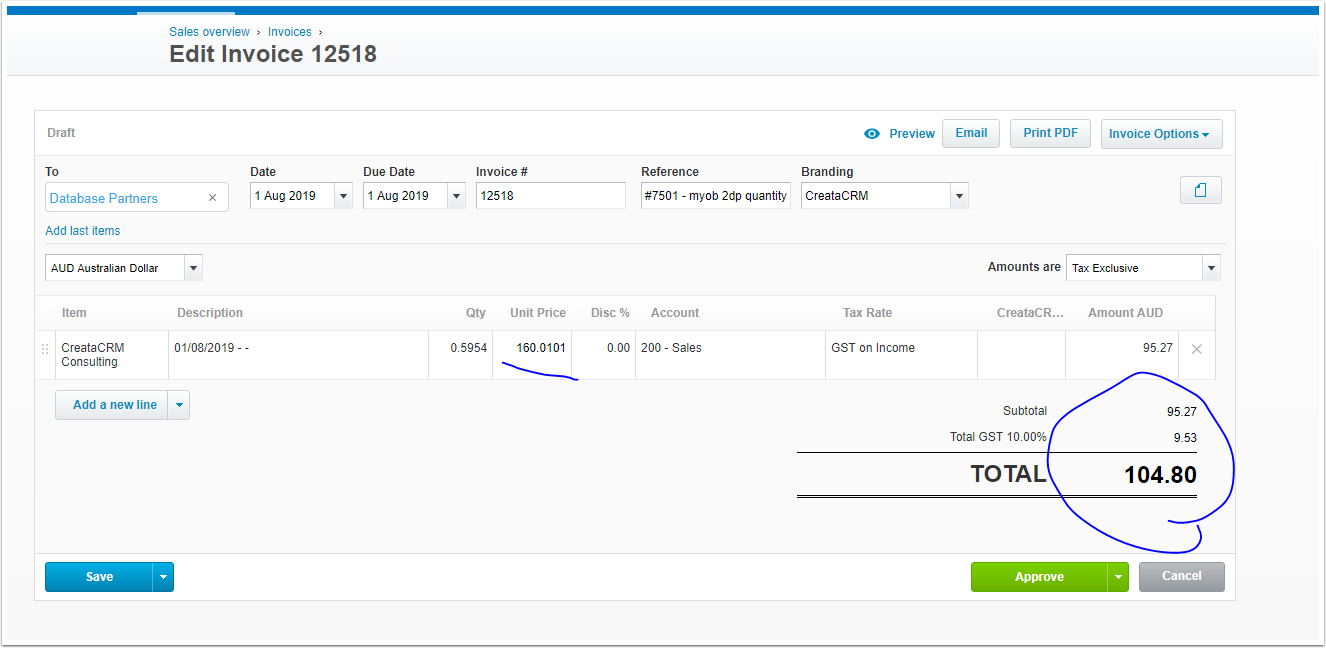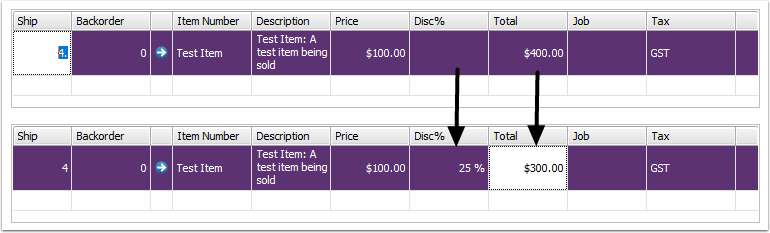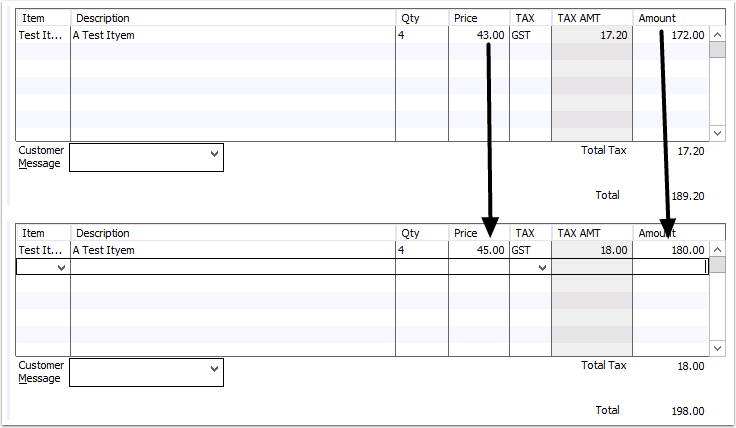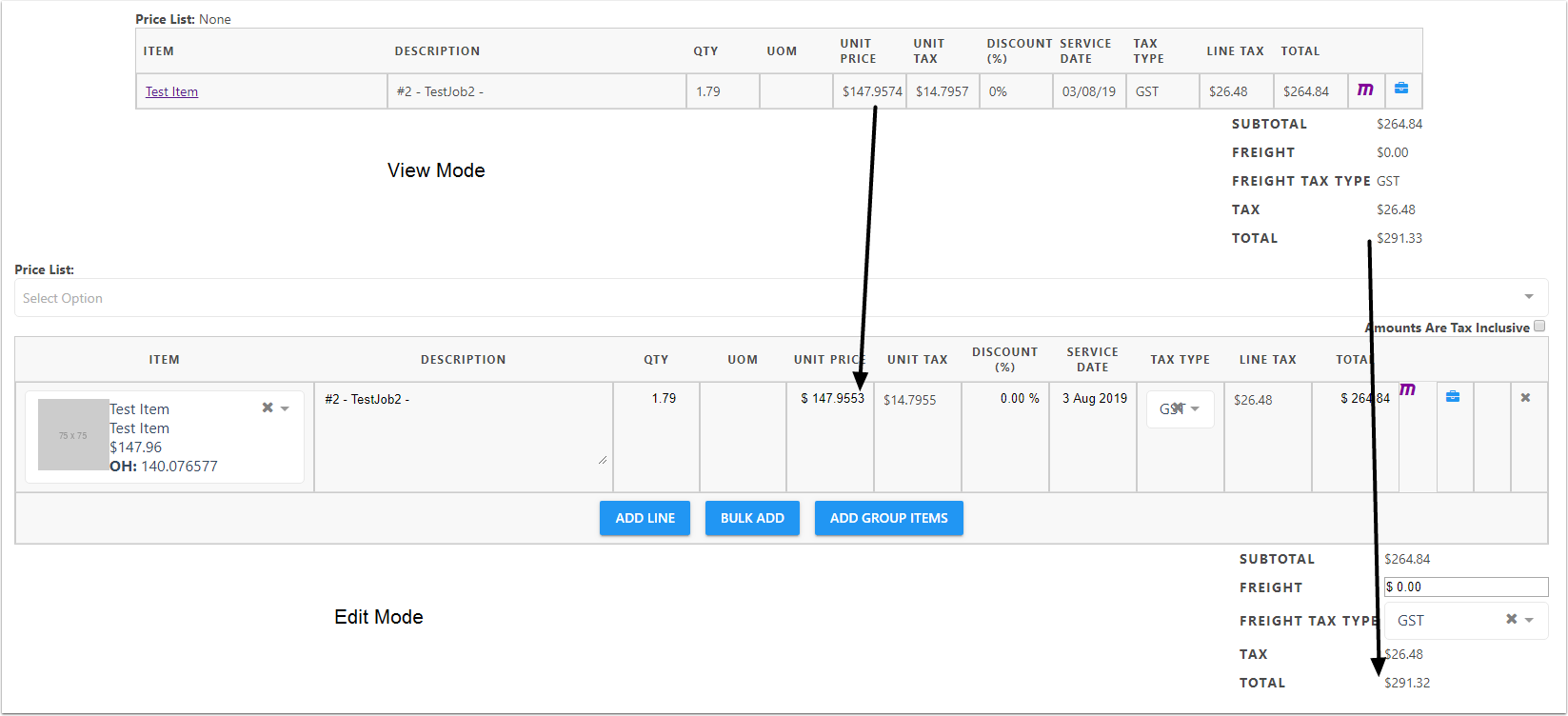# Changing Line editor values

This article describes what happens when the Quantity, Unit Price and Total fields are changed on Invoices, Quotes and Sales Orders inside CreataCRM and how this relates to Acountancy integration.

## In Xero

Xero does not allow the Total (Amount AUD) field of an invoice line to be edited directly, so Xero will modify only the Total field when the Quantity or Unit Price fields are modified.## In MYOB

In MYOB when the Unit Price or Quantity fields are change the Total field also changes to match.

However when the Total field is directly modified MYOB will modify the Discount% so that the calculation is correct.## In Reckon

Reckon will modify the Total when either the Quantity or Price fields are modified.

Reckon will modify the Unit Price to make the calculation correct when the Total is modified.## In CreataCRM

CreataCRM follows the same process that occurs in Reckon:

• If the Quantity or Price fields are modified, the Total will be modified to match.
• If the Total field is modified, the Unit Price will be adjusted to make the calculation correct.

This modification will also occur when you click the edit button on an invoice.

When the system sets up the values in the fields it will first set the Quantity, then the Unit Price, then the Total. If this calculation is not correct, the Unit Price will be adjusted to make so that the calculation is correct.## Edge cases - values not adding up

Occasionally in CreataCRM when you create an invoice you may end up with the below scenario where the Subtotal and the Tax amount do not add up to the Total amount; it may be one cent higher or lower.

This occurs because these fields are rounded; further decimal places for the Subtotal and the Tax field would mean that these individual values will be rounded down, but when added together their sum would be rounded up.

In this case attempting to Edit the invoice will force the system to recalculate these values and so will modify the Total field so that the sum is correct.